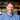# Currying - An Introduction With Function Declarations & Expressions

April 13, 2019

For a long time, I hated seeing functions like this: `const someFn = a => b => a + b;`. I thought this was just “code golf” (the idea of reducing a function to its shortest incarnation) without concern for how it would be received by the reader of the code later.

This can definitely be true and I’m still generally opposed to golf for the sake of itself. But, what I missed was that writing functions in this way, that is - using currying, can actually be really helpful.

Currying enables a greater control over what a function is doing (by reducing each function’s scope) by leveraging the composability of functions.

Let’s start with an example of addition with function declarations. Later, we’ll move into ES6 and function expressions.

The function’s purpose is trivial, but it was this example that helped me see how currying worked!

``````function addOne(a) {
return a + 1
}

return a + b
}

return a + b
}
}``````

If we know we always want to add by one, `addOne` is perfectly reasonable. If we are okay always setting two variables, we can use `addBy`. `addByCurried` seems to be fundamentally different, but it actually allows us to determine what we want to add by separately from our base.

So, we could have the following

``````const addByTwo = addNumsCurried(2)
console.log(

It’s important to note that at the point of assignment, `addByTwo` and `addByThree` are functions.

This is great because it means that we invoke them! We can see this by hopping back into our console and testing it:

``````console.log(addByTwo) //
//    return a + b;
//  }``````

Specifically, they are the function `addBy` which takes a single parameter.

``````addByTwo(3) // 5

Okay, now let’s transition to function expressions and ES6 (for ease of comparison, I’m assuming we’re in a totally new global scope, so we won’t have any name collision issues or previously assigned `const` variables):

``````const addOne = a => a + 1
const addNums = (a, b) => a + b
const addNumsCurried = a => b => a + b``````

Wait, what?

`AddNumsCurried` takes advantage of two syntactic sugar features that arrow functions provide:

1. If there is only one parameter, parentheses (`()`) are optional
2. If the return statement is only one line, there’s an implicit return and braces (`{}`) are not necessary

That means `addNumsCurried` could alternatively be written as:

``````const addNumsCurriedAlt = (a) => {
return (b) => {
return { a + b }
}
}``````

This looks pretty similar to how we had it with function declarations. That’s the point!

What if we take it one step further and use our new adding prowess to the elements of an array?

``````const addOneToEachBasic = ar => ar.map(num => num + 1)

Personally, the difference between `addOneToEachComparatmentalized` and `addOneCurried` is when the light bulb when off! I’d run into this issue a ton with `.reduce` where I wanted to separate my reducer and define it separately, but I always ran into trouble!

It wasn’t until I saw these two side by side producing the same results that I got a better understanding of what was happening.

Let’s throw in a wrinkle: Our array is full of numbers, but they can be represented as strings or numbers (but always one or the other). To check we can use a ternary to check the type. We’ll assign the anonymous function to the variable `ensureNum`.

``````// add type checking to make sure everything is a number
const ensureNum = val => (typeof val == 'string' ? Number(val) : val)``````

We want to do that before we add:

``````const addOneToEachWithType = ar => ar.map(ensureNum).map(num => num + 1)

``````const addByToEachWithType = (ar, by) =>